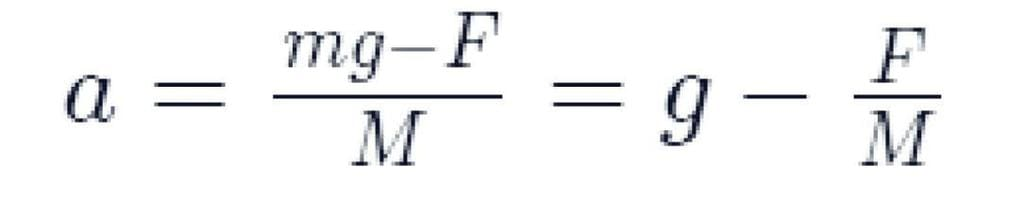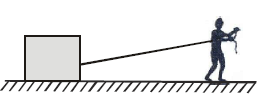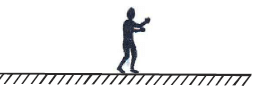NEET  >  Assertion & Reason Test: Laws of Motion

# Assertion & Reason Test: Laws of Motion - NEET

Test Description

## 20 Questions MCQ Test Physics Class 11 - Assertion & Reason Test: Laws of Motion

Assertion & Reason Test: Laws of Motion for NEET 2023 is part of Physics Class 11 preparation. The Assertion & Reason Test: Laws of Motion questions and answers have been prepared according to the NEET exam syllabus.The Assertion & Reason Test: Laws of Motion MCQs are made for NEET 2023 Exam. Find important definitions, questions, notes, meanings, examples, exercises, MCQs and online tests for Assertion & Reason Test: Laws of Motion below.
Solutions of Assertion & Reason Test: Laws of Motion questions in English are available as part of our Physics Class 11 for NEET & Assertion & Reason Test: Laws of Motion solutions in Hindi for Physics Class 11 course. Download more important topics, notes, lectures and mock test series for NEET Exam by signing up for free. Attempt Assertion & Reason Test: Laws of Motion | 20 questions in 40 minutes | Mock test for NEET preparation | Free important questions MCQ to study Physics Class 11 for NEET Exam | Download free PDF with solutions
 1 Crore+ students have signed up on EduRev. Have you?
Assertion & Reason Test: Laws of Motion - Question 1

### Directions : Each of these questions contain two statements, Assertion and Reason. Each of these questions also has four alternative choices, only one of which is the correct answer. You have to select one of the codes (a), (b), (c) and (d) given below.Assertion : On a rainy day, it is difficult to drive a car or bus at high speed.Reason : The value of coefficient of friction is lowered due to wetting of the surface.

Detailed Solution for Assertion & Reason Test: Laws of Motion - Question 1
On a rainy day, the roads are wet. Wetting of roads lowers the coefficient of friction between the types and the road. Therefore, grip on a road of car reduces and thus chances of skidding increases.
Assertion & Reason Test: Laws of Motion - Question 2

### Directions : Each of these questions contain two statements, Assertion and Reason. Each of these questions also has four alternative choices, only one of which is the correct answer. You have to select one of the codes (a), (b), (c) and (d) given below.Assertion : An object can move with constant velocity if no net force acts on it.Reason : No net force is needed to move an object with constant velocity.

Detailed Solution for Assertion & Reason Test: Laws of Motion - Question 2
If assertion is true but reason is false. Velocity of fall becomes constant when downward force due to acceleration is balanced by an upward force of retardation. This constant velocity of an object is known as terminal velocity.
Assertion & Reason Test: Laws of Motion - Question 3

### Directions : Each of these questions contain two statements, Assertion and Reason. Each of these questions also has four alternative choices, only one of which is the correct answer. You have to select one of the codes (a), (b), (c) and (d) given below.Assertion : For the motion of electrons around nucleus, Newton’s second law is used.Reason : Newton’s second law can be used for motion of any object.

Detailed Solution for Assertion & Reason Test: Laws of Motion - Question 3
Newton’s second law can not be used for any object.
Assertion & Reason Test: Laws of Motion - Question 4

Directions : Each of these questions contain two statements, Assertion and Reason. Each of these questions also has four alternative choices, only one of which is the correct answer. You have to select one of the codes (a), (b), (c) and (d) given below.

Assertion : Impulse of force and momentum are same physical quantities.

Reason : Both quantities have same unit.

Detailed Solution for Assertion & Reason Test: Laws of Motion - Question 4
Impulse and momentum are different quantities, but both has same unit (N–s).
Assertion & Reason Test: Laws of Motion - Question 5

Directions : Each of these questions contain two statements, Assertion and Reason. Each of these questions also has four alternative choices, only one of which is the correct answer. You have to select one of the codes (a), (b), (c) and (d) given below.

Assertion : A bullet is fired from a rifle. If the rifle recoils freely, the kinetic energy of rifle is more than that of the bullet.

Reason : In case of rifle bullet system, the law of conservation of momentum violates.

Detailed Solution for Assertion & Reason Test: Laws of Motion - Question 5
The Law of conservation of linear momentum is correct when no external force acts. When bullet is fired from a rifle then both should possess equal momentum but different kinetic energy. Kinetic energy of the rifle is less than that of bullet because E∝1/m. As the mass of the gun is larger, so the kinetic energy of the gun is less than that of bullet.
Assertion & Reason Test: Laws of Motion - Question 6

Directions : Each of these questions contain two statements, Assertion and Reason. Each of these questions also has four alternative choices, only one of which is the correct answer. You have to select one of the codes (a), (b), (c) and (d) given below.

Assertion : The two bodies of masses M and m (M > m) are allowed to fall from the same height if the air resistance for each be the same then both the bodies will reach the earth simultaneously.

Reason : For same air resistance, acceleration of both the bodies will be same.

Detailed Solution for Assertion & Reason Test: Laws of Motion - Question 6
The force acting on the body of mass M are its weight Mg acting vertically downwards and air resistance F acting vertically upward. ∴ Acceleration of the bodyNow, M > m, therefore, the body with larger mass will have greater acceleration and it will reach the ground first.

Assertion & Reason Test: Laws of Motion - Question 7

Directions : Each of these questions contain two statements, Assertion and Reason. Each of these questions also has four alternative choices, only one of which is the correct answer. You have to select one of the codes (a), (b), (c) and (d) given below.

Assertion : Frictional forces are conservating forces.

Reason : Potential energy can be associated with frictional forces.

Detailed Solution for Assertion & Reason Test: Laws of Motion - Question 7
We know that the energy possessed by an object by virtue of its position is the potential energy. Thus, potential energy is associated with conservative only, and since frictional forces are non-conservative forces, potential energy is not associated with them.
Assertion & Reason Test: Laws of Motion - Question 8

Directions : Each of these questions contain two statements, Assertion and Reason. Each of these questions also has four alternative choices, only one of which is the correct answer. You have to select one of the codes (a), (b), (c) and (d) given below.

Assertion: Friction is a necessary evil

Reason: Though friction dissipates power, but without friction we cannot walk.

Detailed Solution for Assertion & Reason Test: Laws of Motion - Question 8
Friction causes wear & tear and loss of energy, so it is an evil but without friction walking. Stopping a vehicle etc. would not be possible. So it is necessary for us.
Assertion & Reason Test: Laws of Motion - Question 9

Directions : Each of these questions contain two statements, Assertion and Reason. Each of these questions also has four alternative choices, only one of which is the correct answer. You have to select one of the codes (a), (b), (c) and (d) given below.

Assertion : A cyclist always bends inwards while negotiating a curve.

Reason : By bending, cyclist lowers his centre of gravity

Detailed Solution for Assertion & Reason Test: Laws of Motion - Question 9
The purpose of bending is to acquire centripetal force for circular motion. By doing so component of normal reaction will counter balance the centrifugal force.
Assertion & Reason Test: Laws of Motion - Question 10

Directions : Each of these questions contain two statements, Assertion and Reason. Each of these questions also has four alternative choices, only one of which is the correct answer. You have to select one of the codes (a), (b), (c) and (d) given below.

Assertion : Linear momentum of a body changes even when it is moving uniformly in a circle.

Reason : In uniform circular motion, velocity remains constant.

Detailed Solution for Assertion & Reason Test: Laws of Motion - Question 10
In uniform circular motion, the direction of motion changes, therefore velocity changes. As P = mv therefore momentum of a body also changes uniform circular motion.
Assertion & Reason Test: Laws of Motion - Question 11

Directions : Each of these questions contain two statements, Assertion and Reason. Each of these questions also has four alternative choices, only one of which is the correct answer. You have to select one of the codes (a), (b), (c) and (d) given below.

Assertion : Force is required to move a body uniformly along a circle.

Reason : When the motion is uniform, acceleration is zero.

Detailed Solution for Assertion & Reason Test: Laws of Motion - Question 11
When a body is moving in a circle, its speed remains same but velocity changes due to change in the direction of motion of body. According to first law of motion, force is required to change the state of a body. As in circular motion the direction of velocity of body is changing so the acceleration cannot be zero. But for a uniform motion acceleration is zero (for rectilinear motion).
Assertion & Reason Test: Laws of Motion - Question 12

Directions : Each of these questions contain two statements, Assertion and Reason. Each of these questions also has four alternative choices, only one of which is the correct answer. You have to select one of the codes (a), (b), (c) and (d) given below.

Assertion: There is a stage when frictional force is not needed at all to provide the necessary centripetal force on a banked road.

Reason: On a banked road, due to its inclination the vehicle tends to remain inwards without any chances of skidding.

Detailed Solution for Assertion & Reason Test: Laws of Motion - Question 12
The assertion is true for a reason that when the car is driven at optimum speed. Then the normal reaction component is enough to provide the centripetal force.
Assertion & Reason Test: Laws of Motion - Question 13

Directions : Each of these questions contain two statements, Assertion and Reason. Each of these questions also has four alternative choices, only one of which is the correct answer. You have to select one of the codes (a), (b), (c) and (d) given below.

Assertion : A man and a block rest on smooth horizontal surface. The man holds a rope which is connected to block. The man cannot move on the horizontal surface.Reason : A man standing at rest on smooth horizontal surface cannot start walking due to absence of friction (The man is only in contact with floor as shown).Detailed Solution for Assertion & Reason Test: Laws of Motion - Question 13
The man can exert force on block by pulling the rope. The tension in rope will make the man move. Hence statement-1 is false.
Assertion & Reason Test: Laws of Motion - Question 14

Directions : Each of these questions contain two statements, Assertion and Reason. Each of these questions also has four alternative choices, only one of which is the correct answer. You have to select one of the codes (a), (b), (c) and (d) given below.

Assertion : A block placed on a table is at rest, because action force cancels the reaction force on the block.

Reason : The net force on the block is zero.

Detailed Solution for Assertion & Reason Test: Laws of Motion - Question 14
The net force on the block is zero, but action cannot cancel the reaction because these two act on different bodies.
Assertion & Reason Test: Laws of Motion - Question 15

Directions : Each of these questions contain two statements, Assertion and Reason. Each of these questions also has four alternative choices, only one of which is the correct answer. You have to select one of the codes (a), (b), (c) and (d) given below.

Assertion: A cricketer moves his hands forward to catch a ball so as to catch it easily without hurting.

Reason: He tries to decrease the distance travelled by the ball so that it hurts less.

Detailed Solution for Assertion & Reason Test: Laws of Motion - Question 15
A cricket player moves his hands backward to increase the time interval for reducing the momentum of the ball to zero. Thus the ball does not hit him hard as force is directly proportional to change of momentum.
Assertion & Reason Test: Laws of Motion - Question 16

Directions : Each of these questions contain two statements, Assertion and Reason. Each of these questions also has four alternative choices, only one of which is the correct answer. You have to select one of the codes (a), (b), (c) and (d) given below.

Assertion: Same force applied for the same time causes the same change in momentum for different bodies

Reason: The total momentum of an isolated system of interacting bodies remains conserved.

Detailed Solution for Assertion & Reason Test: Laws of Motion - Question 16
The momentum of an isolated system of particles is conserved. The forces are internal in nature and sums up to zero for entire system. As the external force is zero on system it's momentum remains conserved.
Assertion & Reason Test: Laws of Motion - Question 17

Directions : Each of these questions contain two statements, Assertion and Reason. Each of these questions also has four alternative choices, only one of which is the correct answer. You have to select one of the codes (a), (b), (c) and (d) given below.

Assertion : Mass is a measure of inertia of the body in linear motion.

Reason : Greater the mass, greater is the force required to change its state of rest or of uniform motion.

Detailed Solution for Assertion & Reason Test: Laws of Motion - Question 17
According to Newton’s second law of motion a = F/m i.e. magnitude of the acceleration produced by a given force is inversely proportional to the mass of the body. Higher is the mass of the body, lesser will be the acceleration produced i.e. mass of the body is a measure of the opposition offered by the body to change a state, when the force is applied i.e. mass of a body is the measure of its inertia.
Assertion & Reason Test: Laws of Motion - Question 18

Directions : Each of these questions contain two statements, Assertion and Reason. Each of these questions also has four alternative choices, only one of which is the correct answer. You have to select one of the codes (a), (b), (c) and (d) given below.

Assertion : If the net external force on the body is zero, then its acceleration is zero.

Reason : Acceleration does not depend on force.

Detailed Solution for Assertion & Reason Test: Laws of Motion - Question 18
The assertion is true by newton second law of motion which state Fnet =ma.

So if Fnet = 0,a = 0. The reason is false as by newton second law Fnet =ma

So acceleration depend on force.

Assertion & Reason Test: Laws of Motion - Question 19

Directions : Each of these questions contain two statements, Assertion and Reason. Each of these questions also has four alternative choices, only one of which is the correct answer. You have to select one of the codes (a), (b), (c) and (d) given below.

Assertion : A rocket works on the principle of conservation of linear momentum.

Reason : Whenever there is change in momentum of one body, the same change occurs in the momentum of the second body of the same system but in the opposite direction.

Detailed Solution for Assertion & Reason Test: Laws of Motion - Question 19
Since here both the assertion and reason are correct but the rocket major principle is Newton's 3rd Law of Motion, where it is irresponsible of motion.
Assertion & Reason Test: Laws of Motion - Question 20

Read the assertion and reason carefully to mark the correct option out of the options given below:

Assertion: Inertia is the property by virtue of which the body is unable to change by itself the state of rest only.

Reason: The bodies do not change their state unless acted upon by an unbalanced external force.

Detailed Solution for Assertion & Reason Test: Laws of Motion - Question 20
Inertia is the property by virtue of which the body is unable to change by itself not only the state of rest, but also the state of motion.

## Physics Class 11

116 videos|451 docs|188 tests
Information about Assertion & Reason Test: Laws of Motion Page
In this test you can find the Exam questions for Assertion & Reason Test: Laws of Motion solved & explained in the simplest way possible. Besides giving Questions and answers for Assertion & Reason Test: Laws of Motion, EduRev gives you an ample number of Online tests for practice

## Physics Class 11

116 videos|451 docs|188 tests

### How to Prepare for NEET

Read our guide to prepare for NEET which is created by Toppers & the best Teachers